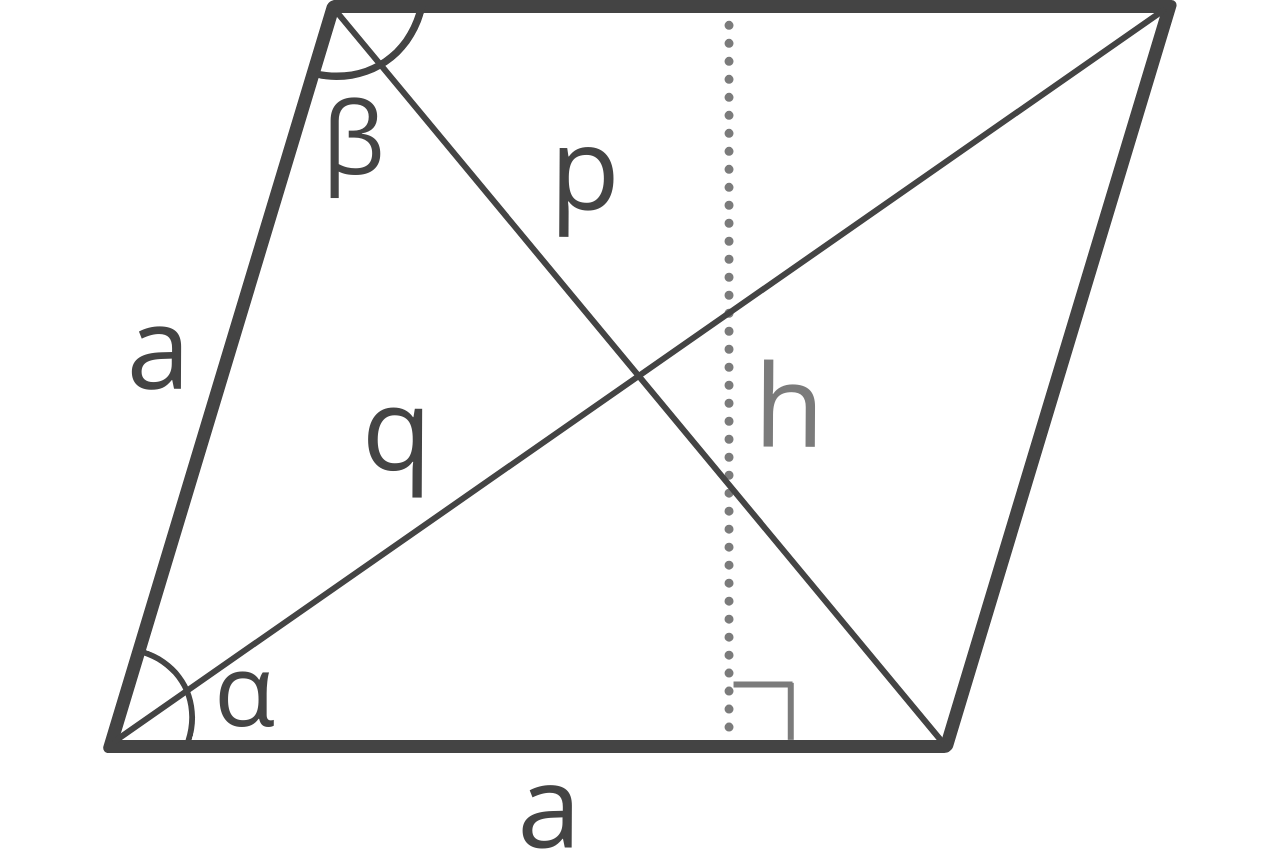# Rhombus Calculator

Use the rhombus calculator below to find all of its properties, including the area, perimeter, diagonals, and angles.## Rhombus Properties:

 Area (A): Perimeter (P): Diagonal (p): Diagonal (q): Angle (α): Angle (β):
Learn how we calculated this below

## How to Find the Properties of a Rhombus

A rhombus is a type of parallelogram, which is distinguished by all four sides being of equal length. Its unique properties include its area, perimeter, angles, and the length of the diagonals.

You can calculate the value of these properties using one of several formulas.

### How to Find the Area of a Rhombus

You can find the area of a rhombus if you know the length of its sides and its height by using the following formula:

A = a \times h

The area A of a rhombus is equal to the length of its sides a times the height h.

### How to Find the Perimeter of a Rhombus

Since a rhombus has four sides of equal length, you can find the perimeter of a rhombus by multiplying the length of the sides by 4.

P = 4a

The perimeter P is equal to 4 times the length of its sides a.

### How to Find the Interior Angles of a Rhombus

A rhombus has four interior angles, two acute and two obtuse. The acute and obtuse angles are supplementary to each other and sum up to 180 degrees.

If you know the length of the sides and height, you can find the angles of a rhombus using the following formulas:

\alpha = sin^{-1}(\frac{h}{a})

The acute angle α of a rhombus is equal to the inverse sine of the height h divided by the length of the sides a.

Since the acute and obtuse angles are supplementary and sum to 180°, you can find the second angle by subtracting the first from 180°.

\beta = 180\degree -\alpha

Since the obtuse angle β is equal to 180° minus the acute angle α.

### How to Find the Diagonals of a Rhombus

You can find the diagonals of a rhombus using the length of the sides and one of the interior angles.

p = a \times \sqrt{2+2cos(\alpha)}
q = a \times \sqrt{2-2cos(\alpha)}

The diagonal p is equal to the length of side a times the square root of 2 plus 2 times the cosine of the angle α. The diagonal q is equal to the length of side a times the square root of 2 minus 2 times the cosine of the angle α.